## Example Questions

2 Next →

### Example Question #11 : How To Find The Volume Of A Cube

Find the volume of a cube whose side length is 0.1.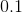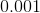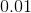Explanation:

To solve, simply use the formula for volume of a cube. The volume of a cube is length times width times height. Since the height, width, and length are equal on a cube the formula becomes side cubed.

We are given the side length of 0.1 thus,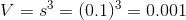### Example Question #11 : How To Find The Volume Of A Cube

Find the volume of a cube given side length is.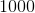Explanation:

To solve, simply cube the side length. Thus,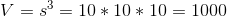2 Next →

### All ACT Math Resources